# Lookup Functions

In this chapter, you will learn one of the most powerful functions Excel has to offer: lookup functions. Specifically, we’ll learn about V Lookup, X Lookup, H Lookup, and Index/Match. These Functions are so important to know that companies looking to hire people that know about Excel will ask specifically about these functions in the interview.

Use this workbook for the chapter.

## Preface

As we go through this practice, we will suppose that we have a student-run company that makes pizzas. There are about a dozen or so ingredients that they use to make those pizzas. We have an ingredient list from the procurement team. From the purchases that the company has made, we have a list of the day we purchased an item, what the item was, and how much we purchased. You will want to add three columns titled Price Per Unit to practice V Lookup, X Lookup, and Index/Match. (See Figure 19.1)

Once we have the price per unit, we can calculate the total cost by multiplying that unit price by the quantity that we purchased so that we can show the finance team on our summary sheet how much it is that we’re spending on a given item and over time.

## VLOOKUP

Let’s get started with the VLOOKUP formula. Use the following steps:

1. Select a cell.
2. Type =VLOOKUP( . (See Figure 19.2)
1. The V stands for vertical lookup.
3. Select the cell that contains the value that you want to look up.
1. For this practice, select Salt. (See Figure 19.2)
1. Excel will next need to know what table array you would like to look in.
5. Select the Ingredient List tab.
1. Notice that Salt is listed in the first column.
1. This means that whatever value you are attempting to look up with the V Lookup function has to be in the first column of your table array.
6. Select the entire table and add another comma
7. Type in the index number.
1. The index in this practice starts with Ingredient as column one, Vendor as column two, Location as column three, and Price Per Unit as column four.
2. Whichever number you type in is the column of the information that will be returned.
3. If we wanted to pull back the price per unit, we need to type in the number 4, because it’s the fourth column in this table.
8. Type False or 0 to get an exact match.
1. The last item is looking for whether you would like to use an approximate match or an exact match.
2. In almost every scenario you encounter, you will want to use an exact match.
9. Close the parentheses.
1. The full function in the function bar should read =VLOOKUP(C3,’Ingredient List’!B2:E14,4,0). (See Figure 19.3)
10. Press Enter
1. Excel will pull back the price per unit of salt at $4.95. If you check the ingredient list, you will see that this value is correct.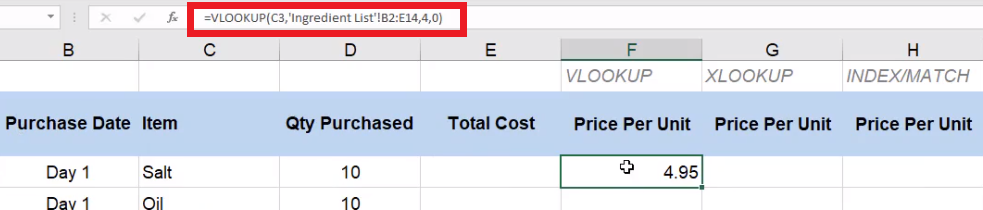Figure 19.3 ## Fixing VLOOKUP Errors If you select and drag the formula down to apply it to the rest of the items in the list, you will notice that some errors start to occur. This is because we did not use anchoring when we created the formula so all of the references are relative references. When we wrote the initial formula, we referenced B2 through E14. However, as the formula was dragged down, another cell will reference a different range, like B6 through E18 When we create these formulas, there are parts that we want to move. For example, we want to change the lookup value as we drag it down each row. However, we want the table array to be consistent when we look it up. In this practice, we also want the column number to stay the same, and we want the range lookup to always be false. If you exclude the false argument, your formula will come back with errors, although those errors will not happen every time. You need to make sure as you create these functions that you’re including that final optional lookup option as false. There are a couple of ways to fix this reference error. One of them is using dollar signs in the formula to make it an absolute reference. In this case, the reference will read !$B$2:$E$14. (See Figure 19.4)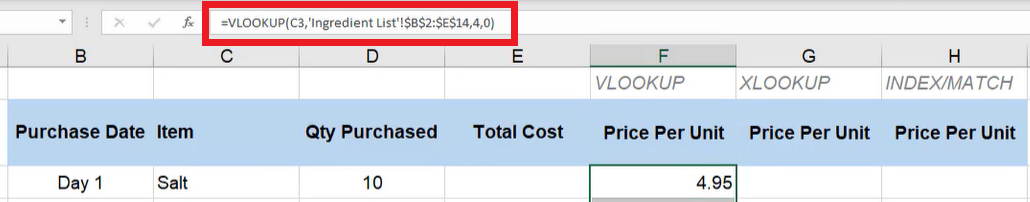Figure 19.4 Then when you drag the formula down, it will reference correctly. Sometimes you may add things to the list after a formula was created. When you try to drag the function down to those added items, they won’t show up in the range. To avoid having to change the formula every time something is added, you can use a named range. For this exercise, use the following steps: 1. Select the table, but stop at the Olive data entry. 1. For this practice, we will pretend that the rest of the list has not been added yet. 2. Select the name of the range and rename it to ing_table. (See Figure 19.5) 1. The ing stands for ingredient 3. Press Enter Once you have done this, go back to the formula. Instead of referencing the long string of B2 through E10 with dollar signs, just type in ing_table. (See Figure 19.5)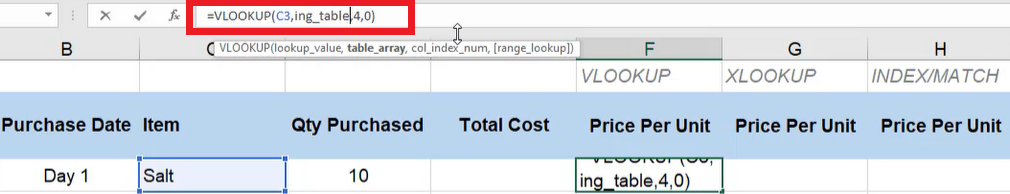Figure 19.5 Now it will always reference that array. You don’t have to worry about anchoring because it is automatically anchored. However, there are additional ingredients not included in that table array. We can fix the issue without having to change the formulas. To do this, use the following steps: 1. Select the Formulas tab. 2. Select Name Manager 1. This will open a dialog box. 3. Select Edit. (See Figure 19.6) 1. This will open a smaller dialog box.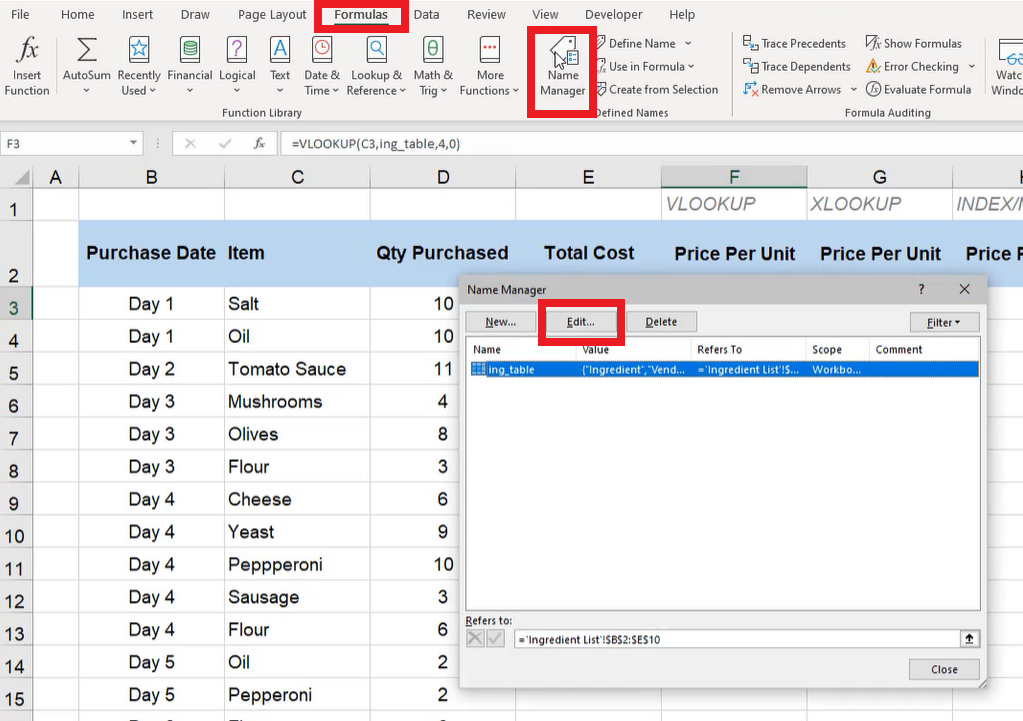Figure 19.6 4. After Refers to:, change$E$10 to$E$14. (See Figure 19.7) 5. Select OK. 1. The formula will now select the entire table. 6. Select Close on the bigger dialog box. 1. The formulas will automatically update. 7. After Refers to:, change$E$10 to$E$14. (See Figure 19.7) 8. Select OK. 1. The formula will now select the entire table. 9. Select Close on the bigger dialog box. 1. The formulas will automatically update.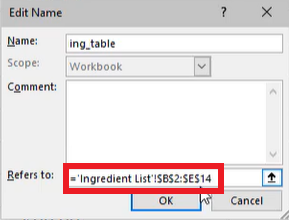Figure 19.7 If you select and drag the formula all the way down the column, Excel’s auto-fill feature will recognize the formula and drag it all the way down. But you may notice that there are still errors. • The function comes back with a N/A because Excel can’t seem to find Yeast on the ingredient list. If you go to the ingredient list, you will notice that, sure enough, Yeast is not one of the items on the list. • Pepperoni is on the ingredient list, but if you look closely at the worksheet that we are calculating VLOOKUP on, Pepperoni is spelled incorrectly. Any spelling mistakes on the data that you’re looking up will cause an error, and it won’t work. • Oil has an extra space after the word left in the cell, which you can find when you select the cell and look at the formula bar. To make sure this error doesn’t occur later without having to search each individual cell, use the following steps: 1. Select the first cell in the column that has the formula applied to it. 2. Add TRIM to the formula, and surround the cell reference with parentheses. (See Figure 19.8 1. In this practice, the cell reference should be C3. 2. The trim function will remove any trailing spaces when Excel does the lookup. 3. Drag the formula down through the rest of the column to apply it to all of those cells. 1. This will correct the error in the data for Oil.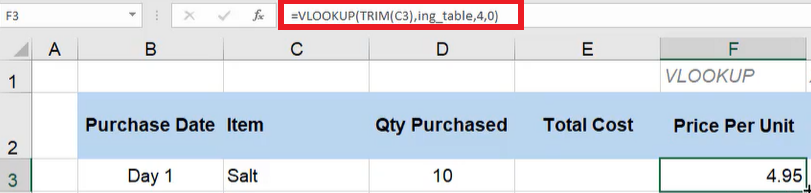Figure 19.8 To fix the issue with Yeast, we need to add it to the ingredient list. 1. Type Yeast in the Ingredient column. 2. Type BYU-Idaho in the Vendor column. 3. Type Rexburg, ID in the Location column. 4. Type$4.78 in the Price Per Unit column.

Add the new row into the named range using the following steps:

1. Select the Formulas tab.
2. Select Name Manager
1. This will open a dialog box.
3. Select Edit. (See Figure 19.6)
1. This will open a smaller dialog box.
4. After Refers to:, change $E$14 to $E$15.

6. Select Close
1. When you go back to the Purchase History sheet, you will see that the error has been fixed.

It’s important to note that we can’t just reference the Ingredient sheet, select the data for salt, and then select and drag it down on the Purchase History sheet. The ingredients are not in the same order in the two lists on each sheet. The items on the Purchase History sheet are randomized by day. You could type the equals sign and reference each respective price per unit for each item, but there is a lot of room for error in that type of work. Instead, we use these lookup functions because they look for the exact thing we are trying to find and pull back the exact value that we need.

## XLOOKUP

The lookup array in XLOOKUP is looking for a single column or a single row. If you go back to the ingredient list, the lookup array is the Ingredient column. The return array, instead of counting the number of columns, is going to be the Price Per Unit array. XLOOKUP is beneficial when the data we are looking up is not in front of what we want to return. For example, you would use XLOOKUP if you wanted to look up the price and return which ingredient it was. Or, you could have it return the vendor, the location, etc. For this practice, change the title under the XLOOKUP column to Vendor. These pieces may not be a unique identifier, so that may not be the best business-use case. It could, however, be an example of how we would use this lookup function. After the lookup array and the return array are put into the function, Excel will ask what you would like it to return if it doesn’t find anything.

To use XLOOKUP, use the following steps:

1. Select the first cell under the XLOOKUP Price Per Unit.
2. Type =XLOOKUP(TRIM(.
1. Excel will ask for a lookup value.
3. Choose Salt.
1. Make sure to close the parentheses for the trim function.
4. Add a comma, and select all of the data in the Price Per Unit column.
5. Add another comma, and select all the data in the Vendor column.
6. Add a comma. Type “Nothing found”.
1. This phrase is what will show up if Excel doesn’t find anything during the lookup.
7. Add a comma and type 0 to get an exact match.
1. We always want to be exact, which, in this formula, is the default.
8. Leave the search mode empty. Our data is not long enough for this part of the function to matter.
1. If it is a large set of data, the search mode will give Excel some hints on where it might find what you’re looking for. This will help the lookup go faster with larger data sets.
9. Close the parentheses.For this exercise, the formula bar should read as follows: =XLOOKUP(TRIM(C3),‘Ingredient List’!E3:E15,’Ingredient List’!C3:C15,”Nothing found”,0).
10. Press Enter.

You will notice that Excel pulls up Nothing found. This is because the initial lookup value was to try to find the ingredient. To reverse engineer the formula to find the Price per unit instead of the ingredient, use the following steps:

1. Highlight TRIM(C3) in the formula. (See Figure 19.10)
2. Select the Price Per Unit you want to look up.
1. For this exercise, select 4.95 in the Salt row.
3. Press Enter
1. Excel will return with the vendor name (Norton).

As you drag the formula down the column, you will notice that you will run into the same issue of the lists not being anchored. You can fix this by following these instructions:

1. Name the Price Per Unit range price
2. Name the Vendor range vendor
3. In the formula bar, change ‘Ingredient List’!E3:E15 to price.
4. In the formula bar, change ‘Ingredient List’!C3:C15 to vendor
5. The formula should now read =XLOOKUP(F3,price,vendor,”Nothing found”,0). (See Figure 19.11
6. Press Enter

You can now drag the formula down the column, and Excel will fill it in with no issues.

## Index and Match

Index and match is actually a method to look things up using two different functions embedded within each other. We’ll start with the index function. First, the index function asks what array you would like it to return. In this practice, we are going to choose a new array to return: location. Then, the index function will ask you what row number to return, so we’ll use the embedded function Match. Match first wants to know what value to look up. For this practice, we want to look up the item within the array of the Item column on the Purchase History worksheet.

To use Index and Match for this practice, use the following steps:

1. Type =INDEX(.
2. Select the data in the Location column.
3. Add a comma and type MATCH(.
4. Go to the Purchase History worksheet and select Salt.
5. Add a comma, and select all the data under the Ingredient column of the Ingredient list.
6. Add a comma, and type 0 to make the match type exact.
7. Add two parentheses at the end to end both the Match and the Index functions.
1. The formula bar should read =INDEX(‘Ingredient List’!D3:D15,MATCH(‘Purchase History’!C3,’Ingredient List’!B3:B15,0)). (See Figure 19.12)
8. Press Enter.
1. The function should bring back Lubbock, TX.

There are a few improvements needed in this function. Use the following steps to do so:

1. Use the TRIM function to fix the Match data.
1. Type TRIM after the open parenthesis in the Match function.
2. Rename the data under the Location column location.
3. Rename the data in the Ingredient column ing.
4. In the formula bar, change ‘Ingredient List’!D3:D15 to location
5. Change ‘Ingredient List!B3:B15 to ing. (See Figure 19.13)
6. Press Enter
1. As you drag the formula down through the column, Excel will return with each of the item’s locations.
2. Change the title of the column under Index/Match to Vendor Location.

The final piece of this worksheet will be to input the total cost calculation. The total cost calculation is the quantity purchased multiplied by our price unit.

1. Select the first cell under Total Cost.
2. Type the equals ( = ) sign and select the first cell under Qty Purchased.
3. Type an asterisk ( * ).
4. Select the first cell under Price Per Unit.
1. The formula should read =D3*F3. (See Figure 19.14)
5. Press Enter.
1. Excel should return with 49.5

Select all of the data under Total Cost and Price Per Unit and put it into currency format (for PC and Mac use Control + Shift + 4). The summary sheet will have two input cells: Ingredient and Information. Use the following steps to complete a data validation for Ingredients:

1. Select the cell to the right of Ingredient.
2. Select the Data tab.
3. Select Data Validation. (See Figure 19.15

4. In the Allow dropdown bar, choose List
5. Under Source, type =ing to signal the named range for the Ingredient column. (See Figure 19.16

6. Select OK
1. Excel will make the selected cell a dropdown list that allows you to choose an ingredient from the list. (See Figure 19.17)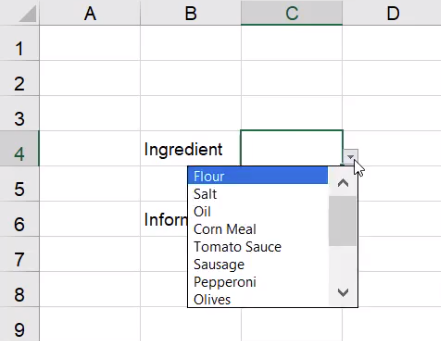Figure 19.17

7. On the Home tab, use the Input cell style. (See Figure 19.18)

We’ll also use data validation in the Information cells. To do so, use the following steps:

1. Select the cell to the right of Information
2. Select the Data tab.
3. Select Data Validation
1. A dialog box will appear.
4. In the Allow dropdown bar, choose List.

6. Go to the ingredient list, and choose Vendor, Location, and Price Per Unit
7. Press the arrow again. (See Figure 19.20)
8. Select OK.

## HLOOKUP

Next, we’ll use HLOOKUP. The H stands for Horizontal Lookup. To use HLOOKUP, complete the following steps:

1. Type Result into another cell on the Summary worksheet.
2. Format the cell to the right of it with the Output style. (See Figure 19.21)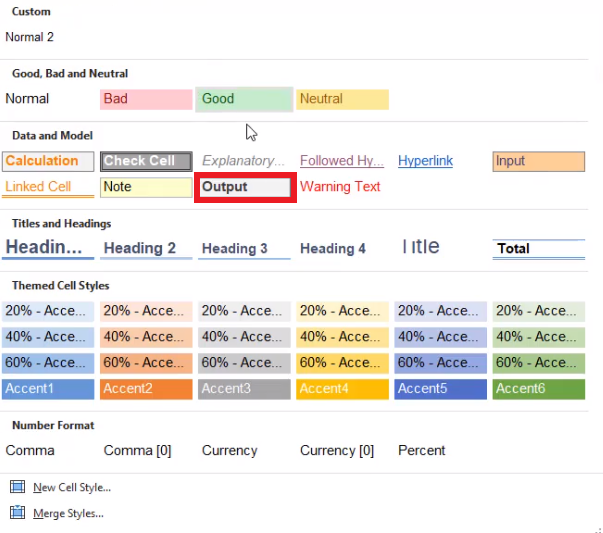Figure 19.21

3. Type =HLOOKUP(.
4. Select the Location cell.
5. Add a comma, and select the entire table on the Ingredient List worksheet.
1. Excel will automatically remember the name of this range as ing_table.
6. Add a comma, and type in the row index number.
1. This refers to which row down you want Excel to go to.
2. In this case, let’s assume we want the row for Flour. This is the second row down on the table, so type 2.
7. Add a comma, and type 0 to get an exact match.
8. Press Enter.
1. Excel will pull back the location in the second row of the Ingredient List table, which is Lansing, MI.

Next, let’s pull back the location for the tomato sauce vendor. To do this, complete the following:

1. Include Tomato Sauce in the row index number in the formula.
2. Select the formula next to Result.
3. Remove the 2 in the row index number slot in the formula. (See Figure 19.22)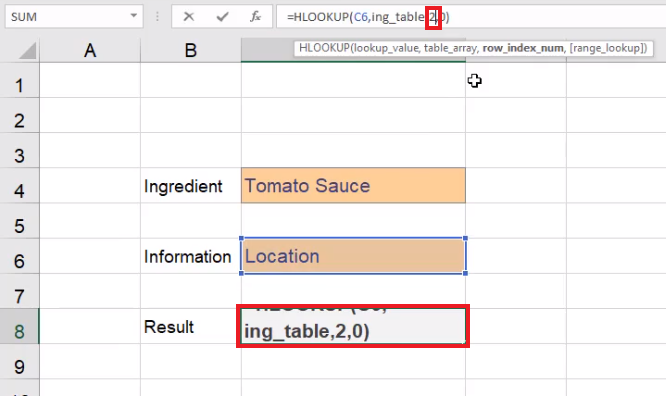Figure 19.22

4. Replace the 2 with a Match function by typing MATCH(.
5. Select Tomato Sauce next to Ingredient. This is our lookup value.
6. Add a comma, and select the entire Ingredient column in the Ingredient List page. This is the lookup array.
7. Add another comma, and type 0 for the match type.
8. Close the parentheses.
9. Press Enter
1. Excel will pull up the location for Tomato Sauce, which is Sacramento, California.
2. The full formula should read =HLOOKUP(C6,ing_table,MATCH(C4,’Ingredient List’!B2:B15,0),0).

If you wanted the vendor name instead, use the following steps:

1. Select the formula cell next to Information, then select the arrow on the right of the cell.
1. A drop-down menu should appear.
2. Select Vendor. (See Figure 19.23)
1. Excel will move the result one cell to the left on the Ingredient List sheet and pull up the vendor for Tomato Sauce, Hintz.

## Finishing the Summary Sheet

Let’s finish up our summary sheet. Using the lookup function, we may want to have a static ability to pull back the total cost of items we have purchased. To do this, complete the following:

1. Add a column to the Ingredient List chart and title it Total Cost
2. Format the Total Cost column like the Price Per Unit column.
1. Select the entire Price Per Unit column.
2. Select the Format Painter. (See Figure 19.24
3. Select the entire Total Cost column. The format painter will copy the format from Price Per Unit and apply it to the new Total Cost column.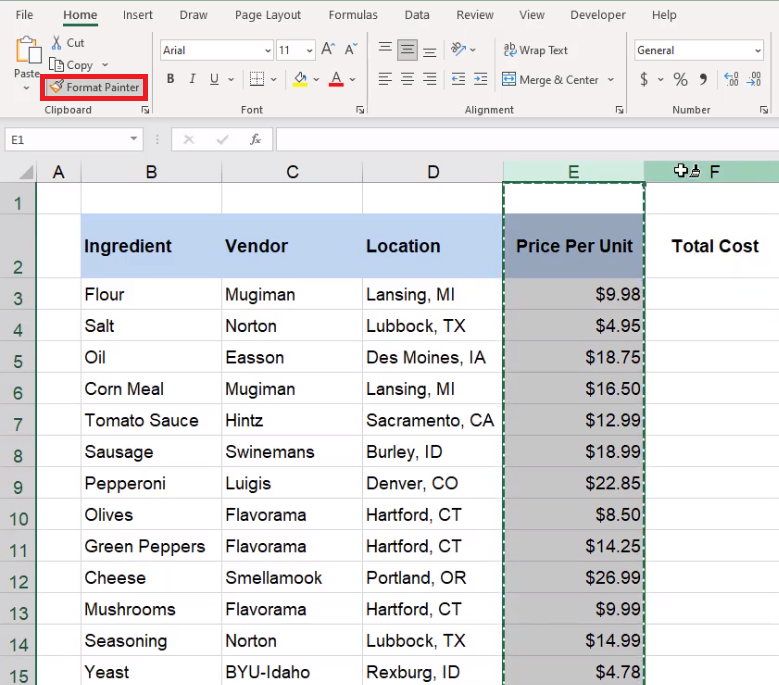Figure 19.24

Next, we will use the SUMIFS formula. To do this complete the following steps:

1. Select the first cell under Total Cost.
2. Type =SUMIFS(.
3. Go to the Purchase History sheet and select the entire Total Cost column.
1. This should be Column E.
2. This is the sum range.
4. Add a comma, and select the entire Item column.
1. This should be Column C.
2. This is the criteria range.
5. Add a comma, go back to the Ingredient List sheet, and select Flour.
1. This is the criteria.
6. Close the parentheses. The whole formula should read =SUMIFS(‘Purchase History’!E:E,’Purchase History’!C:C,’Ingredient List’!B3).
7. Press Enter.
1. Excel will pull up the total cost for Flour.
8. Drag the formula down the rest of the column to apply it to the rest of the ingredients in the table.

Let’s finish up the Summary sheet by including the total cost. To do this, complete the following steps:

1. On the Summary sheet, select a cell under Result and type Total Cost.
2. In the cell to the right of Total Cost, add three lookup functions.
1. VLOOKUP
2. XLOOKUP
3. INDEX/MATCH
3. Format the three cells to the left of the lookup functions as Outputs. (See Figure 19.25)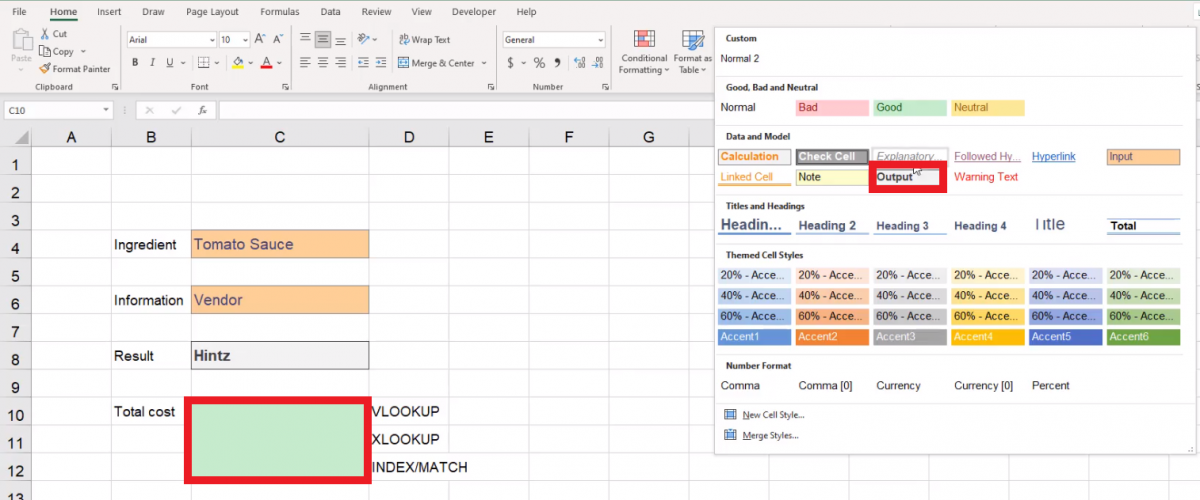Figure 19.25

4. Format the lookup types as Explanatory Notes. (See Figure 19.26)

5. Above all of the information, type Pizza Company Lookup
1. Format it across the top as a heading, and adjust the row height accordingly.
2. Hide the rest of the columns and rows that are not part of this data.
6. Go to the View tab and uncheck the Gridlines checkbox to turn off the ability to see gridlines. (See Figure 19.27)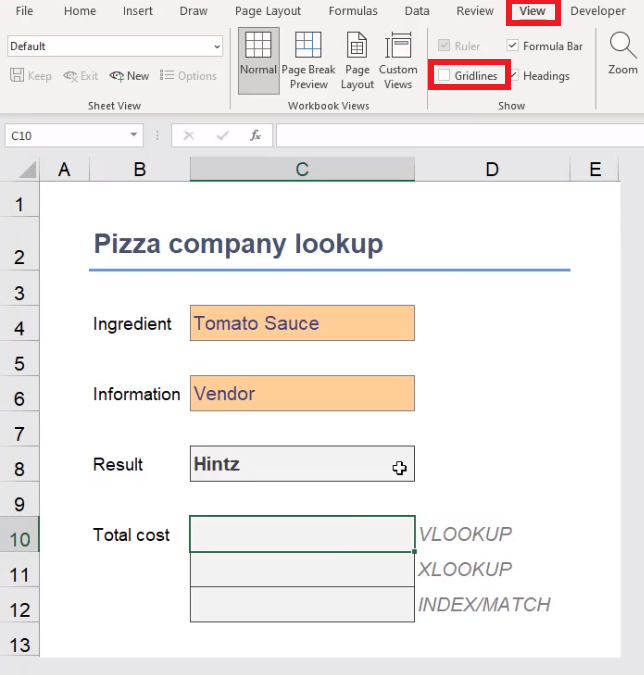Figure 19.27

Let’s formulate the VLOOKUP in the Total Cost. Complete the following steps:

1. Select the output-formatted cell to the left of the VLOOKUP label.
2. Type =VLOOKUP(.
3. Select the Ingredient input box.
1. It should still say “Tomato Sauce.”
2. This is the value for the formula.
4. Add a comma. On the Ingredient List sheet, select the entire table.
1. This is the table array.
2. Remember that we have added a column, so we cannot use our named range this time.
1. This is the column index number.
1. This is the range lookup, and it should always be false.
7. Close the parentheses. The final formula should read as follows: =VLOOKUP(C4.’Ingredient List’!B2:F15,5,0)
8. Press Enter.

Let’s formulate the XLOOKUP in the Total Cost. Complete the following steps:

1. Select the output-formatted cell to the left of the XLOOKUP label.
2. Type =XLOOKUP(.
3. Select the Ingredient input box.
1. This is the value for the formula.
4. Add a comma, and select the data in the Ingredient column on the Ingredient List sheet.
1. This is the lookup array.
5. Add a comma, and select the data in the Total Cost column on the Ingredient List sheet.
1. This is the return array.
1. This will display if the value you are trying to look up could not be found.
7. Add a comma, and type 0 for an exact match.
9. Press Enter

Let’s formulate the Index/Match Total Cost Formula. Complete the following steps:

1. Select the output-formatted cell to the left of the INDEX/MATCH label.
2. Type =INDEX(.
3. Go to the Ingredient List sheet and select the data in the Total Cost column.
1. Remember that Index starts with the array you would like to return.
4. Add a comma, and type MATCH(.
1. This will help the formula find the right row number.
5. Add a comma. On the Summary sheet, select Tomato Sauce.
1. This is the lookup value.
6. Add a comma. On the Ingredient List, select the data in the Ingredient column.
1. This is the lookup array.
8. Close both sets of parentheses. The final formula should read as follows: =INDEX(‘Ingredient List’!F3:F15,MATCH(Summary!C4,ing,0))
9. Press Enter.

You will see that these three formulas illustrate three ways to get the same type of information. Lookup functions are some of the most valuable and powerful functions that Excel has to offer because you can combine information across different worksheets, either on a dashboard or within embedded data tables. You will need to practice these in order to get the hang of how they work and to be confident in correcting errors that come up. Over the course of the projects and practices that we do, please try out using the different methods that have been demonstrated here in this chapter so that you can be comfortable with any and all of them.

### End-of-Chapter Survey

: How would you rate the overall quality of this chapter?
1. Very Low Quality
2. Low Quality
3. Moderate Quality
4. High Quality
5. Very High Quality
Comments will be automatically submitted when you navigate away from the page.